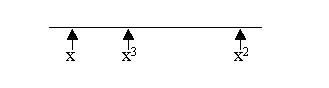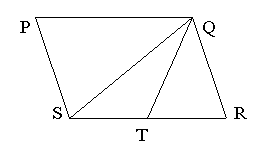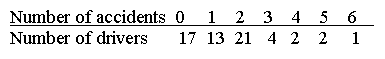## GMAT - Problem Solving - Test 6

A. 1
B. 2
C. 3
D. 4
E. 5

#### 2. What is the greatest of 3 consecutive integers whose sum is 24 ?

A. 6
B. 7
C. 8
D. 9
E. 10A. 5/3
B. 3/5
C. -2/5
D. -5/2
E. none

A. 8
B. 9
C. 10
D. 11
E. 12

A. 20
B. 60
C. 100
D. 110
E. 120

#### 6. 6 pints of a 20 percent solution of alcohol in water are mixed with 4 pints of a 10 percent alcohol in water solution. The percentage alcohol in the new solution is

A. 16
B. 15
C. 14
D. 13
E. 12#### 7. PQRS is a parallelogram and ST = TR. What is the ratio of the area of triangle QST to the area of the parallelogram?

A. 1 : 2
B. 1 : 3
C. 1 : 4
D. 1 : 5
E. it cannot be determined

#### 8. A picture is copied onto a sheet of paper 8.5 inches by 10 inches. A 1.5 inch margin is left all around. What area in square inches does the picture cover?

A. 76
B. 65
C. 59.5
D. 49
E. 38.5A. 0.5
B. 1
C. 1.5
D. 2
E. 4

A. Vr / (12 - V)
B. Vr + V /12
C. Vr - 12
D. V / r - 12
E. V (r + 1) /12